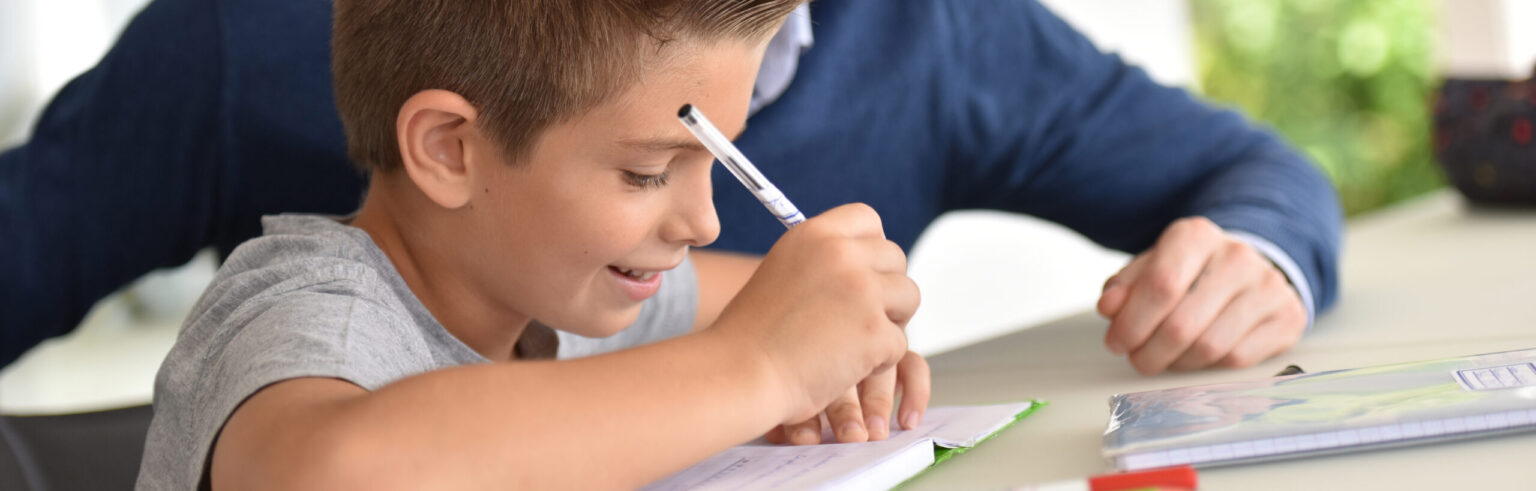# What is the only number that is exactly twice the sum of its digits?Had a look at the question? Got a paper and pen handy? Let’s take a look at the answer!

First:

Our number has digits, so it must be 10 or greater.

Second:

Our number cannot be greater than 36 (since the maximum value of each digit is 9, and 2 x (9 + 9) = 36.

Third:

Our number must be even (since it’s been doubled.)

So, a search of our even numbers between 10 and 36 yields the solution 18. Alternatively, the algebraic solution can be written as: Let the first digit be ‘a’ and the second digit be ‘b’:

10a + b  =  2(a + b)

10a + b  =  2a + 2b

8a        =   b

Since  a and b are digits, they can only take whole number values between 0 and 9 – this makes the only valid solution to be a = 1 and b = 8. The algebraic solution has the advantage that it is easily applied to the extension of this problem, i.e. three times, four times, five times or n times the sum of its digits.

Can you find the only value of n (between 2 and 9) that has a multiple solution?

Related posts

# Are you a parent, teacher or student?

Are you a parent or teacher?

## Hi there!

Book a chat with our team